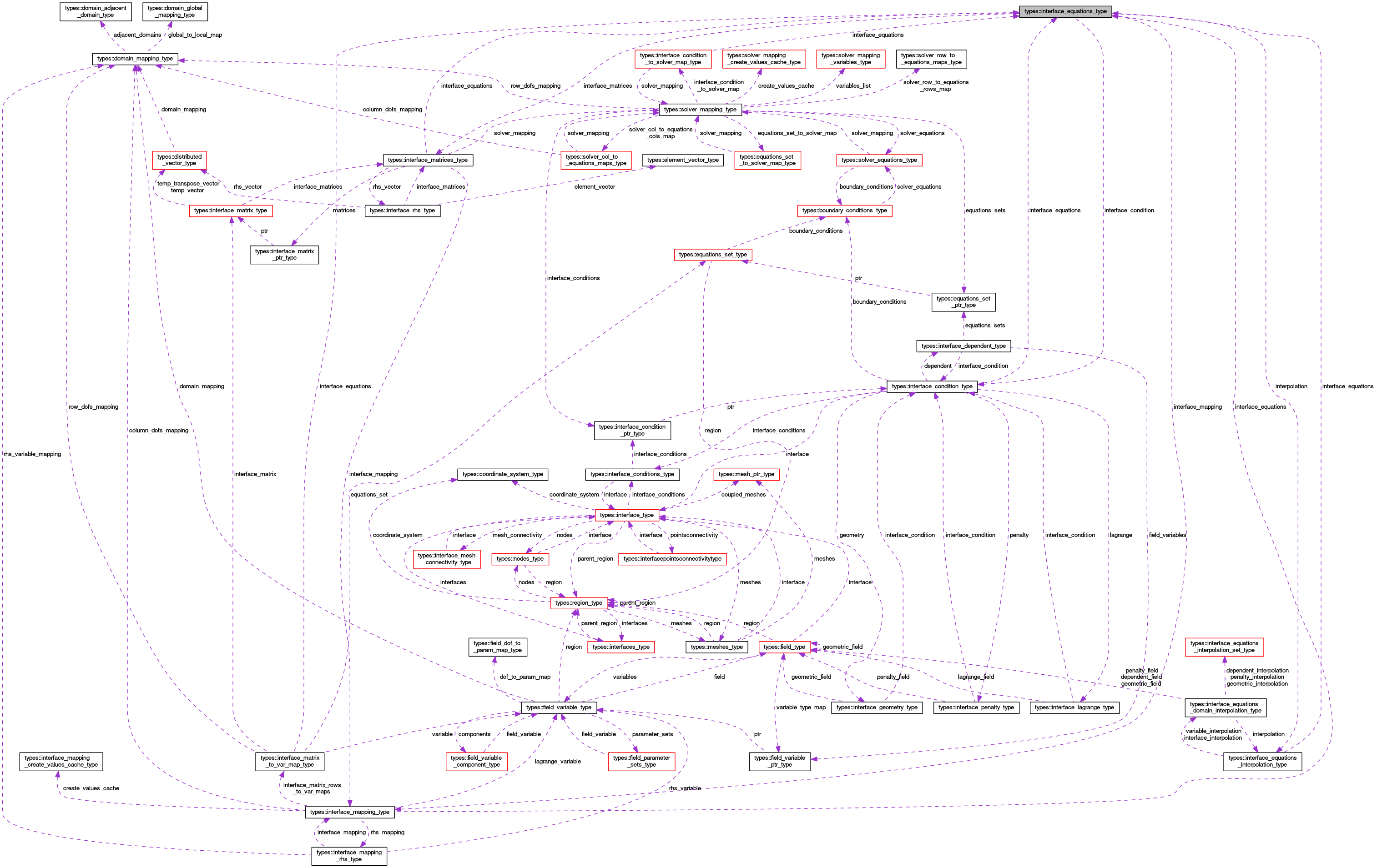OpenCMISS-Iron Internal API Documentation
types::interface_equations_type Type Reference

Contains information about the interface equations for an interface condition. More...

Collaboration diagram for types::interface_equations_type:[legend]

## Public Attributes

type(interface_condition_type), pointer interface_condition
A pointer to the interface condition. More...

logical interface_equations_finished
Is .TRUE. if the interface equations have finished being created, .FALSE. if not. More...

integer(intg) output_type
The output type for the interface equations. More...

integer(intg) sparsity_type
The sparsity type for the interface equation matrices of the interface equations. More...

integer(intg) linearity
The interface equations linearity type. More...

integer(intg) time_dependence
The interface equations time dependence type. More...

type(interface_equations_interpolation_type), pointer interpolation
A pointer to the interpolation information used in the interface equations. More...

type(interface_mapping_type), pointer interface_mapping
A pointer to the interface equations mapping for the interface. More...

type(interface_matrices_type), pointer interface_matrices
A pointer to the interface equations matrices and vectors used for the interface equations. More...

## Detailed Description

Contains information about the interface equations for an interface condition.

Definition at line 2110 of file types.f90.

## Member Data Documentation

 type(interface_condition_type), pointer types::interface_equations_type::interface_condition

A pointer to the interface condition.

Definition at line 2111 of file types.f90.

 logical types::interface_equations_type::interface_equations_finished

Is .TRUE. if the interface equations have finished being created, .FALSE. if not.

Definition at line 2112 of file types.f90.

 type(interface_mapping_type), pointer types::interface_equations_type::interface_mapping

A pointer to the interface equations mapping for the interface.

Definition at line 2118 of file types.f90.

 type(interface_matrices_type), pointer types::interface_equations_type::interface_matrices

A pointer to the interface equations matrices and vectors used for the interface equations.

Definition at line 2119 of file types.f90.

 type(interface_equations_interpolation_type), pointer types::interface_equations_type::interpolation

A pointer to the interpolation information used in the interface equations.

Definition at line 2117 of file types.f90.

 integer(intg) types::interface_equations_type::linearity

The interface equations linearity type.

INTERFACE_CONDITIONS_CONSTANTS::LinearityTypes,INTERFACE_CONDITIONS_CONSTANTS

Definition at line 2115 of file types.f90.

 integer(intg) types::interface_equations_type::output_type

The output type for the interface equations.

INTERFACE_EQUATIONS_ROUTINES::OutputTypes,INTERFACE_EQUATIONS_ROUTINES

Definition at line 2113 of file types.f90.

 integer(intg) types::interface_equations_type::sparsity_type

The sparsity type for the interface equation matrices of the interface equations.